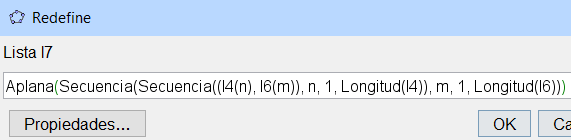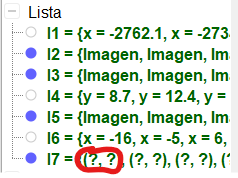Cecilia Lucía Gigena shared this question 2 years ago

Hi,

I'm entering this, and it is not working:

Aplana(Secuencia(Secuencia((l4(n), l6(m)), n, 0, Longitud(l4)), m, 0, Longitud(l6)))

I4 and I6 are sequences of LINES

I pretend to associate lines as a sort of grid, in order to use them to reflect an object in every single space (all along x and all along y).

I've already succesfully done this list:

Secuencia(Refleja(imagen6, l4(n)), n, 0, Longitud(l4), 1)

Which reflects imagen6 all along every line that belongs to list l4.

I want to reflect every reflected image also with every element of list I6, so I that I have length(l6)*length(l4) reflections. This will cover a large area and simulate a continuous background.

I provide a dropbox link in case you cannot open my attachment: https://www.dropbox.com/s/e...1

```Flatten(Sequence(Sequence((l4(n), l6(m)), n, 0, Length(l4)), m, 0, Length(l6)))
Sequence(Reflect(imagen6, l4(n)), n, 0, Length(l4), 1)
```1

In GeoGebra, lists start from 1 not zero so try

`Flatten(Sequence(Sequence((l4(n), l6(m)), n, 1, Length(l4)), m, 1, Length(l6))) `1

Still not working :(1

(line,line) is bad syntax. Maybe you mean this. If not please say what output you want exactly :)

```Flatten(Sequence(Sequence(Intersect(l4(n), l6(m)), n, 1, Length(l4)), m, 1, Length(l6)))
```1

Oh I see. Yes, I wanted line line. I don't know if there's any other way to do this...

I have a list of IMAGES, which are all reflected from a source image with a list of lines, all parallel to x axis.. So it's something like: reflect image in line 1, reflect image in line 2, reflect image in line 3... etc. I want to ALSO reflect each one of this reflections in each one of another list of lines, which are all parallel to y axis. so that they provide a sort of grid: image is reflected in line_y_1 producing image_1, then image is reflected in line_x_1 producing image_2, then image_1 is also reflected in in line_x_1 producing image_3... etc.

I tried to use the image list and syntax didn't work. So... any ideas?1

(line,line) no representa nada pues es interpretado como un punto con ecuaciones de coordenadas. escribelo entre llaves {line,line} flatten es un comando para listas1

Si, tengo dos listas de líneas, quiero reflejar una imagen en todas las líneas de la lista 1 y a su vez todos los reflejos reflejarlos en todas las líneas de la lista 2, por eso quería usar flatten, es un entramado para cubrir toda una superficie con repeticiones del mismo dibujo1

In that case you should use Sequence(Sequence(Translate(...1

What's the syntax for that?

I've tried Zip, but it does not sort all values in list 1 with all values in list 2, it just makes a sort of linear function where x is in list 1 and y is in list 2